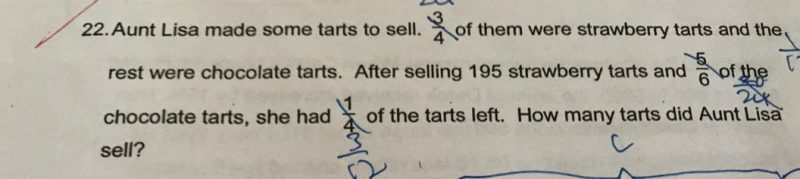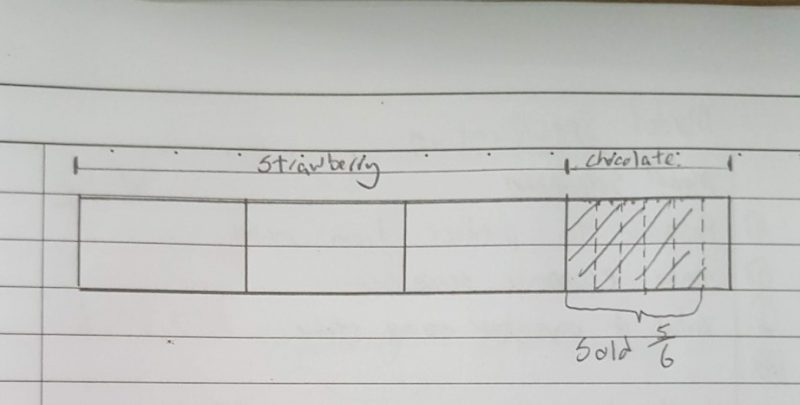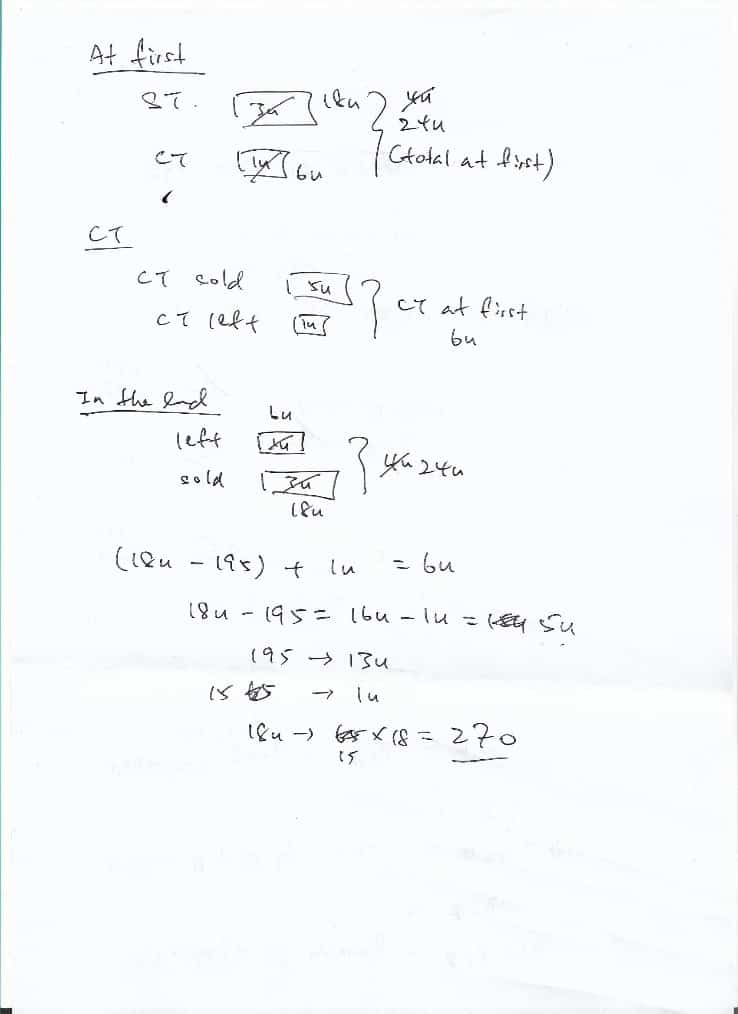# QuestionHi,

Kindly help on this question.

Thank youAfter selling 195 strawberry tarts and 5/6 chocolate tarts she had 1/4 left.

Meaning, strawberry tarts need to be left with 5/6 so that when add with 1/6 of chocolate it will be 1/4.

Cutting each unit of strawberry tarts into 6 unit each we have a total of 18 units.

Left with 5 unit meaning, She sold 13 units, which is equal to 195 strawberry tarts.

1 unit = 195 / 13 = 15

5 units = 15 * 5 = 75 (chocolate tarts sold)

Total sold = 75 + 195 = 270 Tarts

Steps

Total Strawberry Tarts = 3/4 * 6/6                                                                                                                                                                = 18/24

Total Tarts Left = 1/4 * 6/6                                                                                                                                                                 = 6/24

Strawberry Tarts left = 6/24 – 1/24                                                                                                                                                             = 5/24

Strawberry Tarts Sold = 18/24 – 5/24                                                                                                                                                         = 13/24

13/24 = 195

1/24 = 195 / 13                                                                                                                                                                    = 15

18/24 = 15 * 18                                                                                                                                                                      = 270 Tarts Sold

0 Replies 1 Like ✔Accepted Answer0 Replies 0 Likes

S     : C  : Total
3u   : 1u  : 4u
18u : 6u : 24u

CS : CL : C
5u  : 1u   : 6u

SS : SL : S
195 : SL  : 18u

Given CL + SL = ¼ of tarts, or  6u
1u + SL = 6u
SL = 5u

195 + SL = 18u
18u-5u = 195
13u = 195
1u = 15
5u = 75   (CS)

Total tarts sold –> CS + SS = 75 + 195
= 270 (Ans)

0 Replies 1 Like